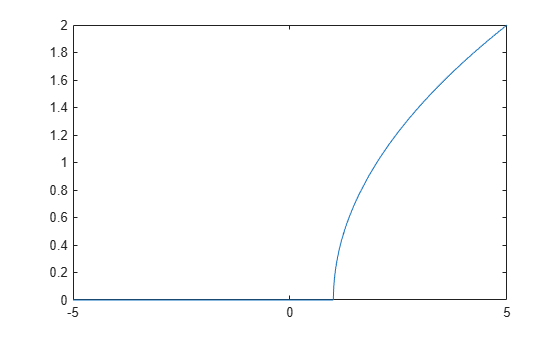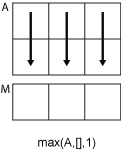# max

Maximum elements of symbolic input

## Syntax

``M = max(A)``
``M = max(A,[],nanflag)``
``M = max(A,[],dim)``
``M = max(A,[],dim,nanflag)``
``M = max(A,[],'all')``
``M = max(A,[],'all',nanflag)``
``C = max(A,B)``
``C = max(A,B,nanflag)``

## Description

example

````M = max(A)` returns the maximum elements of a symbolic input. If `A` is a vector, then `max(A)` returns the maximum of `A`.If `A` is a matrix, then `max(A)` is a row vector containing the maximum value of each column. For an input `A` that contains symbolic expression, the symbolic `max` function returns an unevaluated expression that is reduced by eliminating arguments that do not represent maximum values. The output may have another argument that represents the condition for the symbolic variable. For example, `syms x; max([1 x])` returns the output ```max([1, x], [], 2, 'Omitnan', ~in(x, 'real'))``` in the Command Window since `x` is complex.```

example

````M = max(A,[],nanflag)` specifies whether to include or omit `NaN` values in the calculation. For example, `max(A,[],'includenan')` includes all `NaN` values in `A` while `max(A,[],'omitnan')` ignores them.```

example

````M = max(A,[],dim)` returns the maximum element along dimension `dim`. For example, if `A` is a matrix, then `max(A,[],2)` is a column vector containing the maximum value of each row.```
````M = max(A,[],dim,nanflag)` also specifies the dimension to operate along when using the `nanflag` option.```

example

````M = max(A,[],'all')` returns the maximum over all elements of `A`.```
````M = max(A,[],'all',nanflag)` computes the maximum over all elements of `A` when using the `nanflag` option.```

example

````C = max(A,B)` returns an array with the largest elements taken from `A` or `B`.```
````C = max(A,B,nanflag)` also specifies how to treat `NaN` values.```

## Examples

collapse all

Create a symbolic vector of real elements. Find the largest real element using the symbolic `max` function.

```syms x real A = [23 42 37 18 x]; M = max(A)```
`M = $\mathrm{max}\left(\left[42,x\right],\left[\right],2,\mathrm{"omitnan"},\mathrm{symfalse}\right)$`

The symbolic `max` function returns an unevaluated expression. The expression is reduced by eliminating arguments that do not represent maximum values.

Create a symbolic vector and compute its maximum, excluding `NaN` values.

```syms x positive A = [1.75 x 3.25 -2.5 NaN 0.5 NaN 0.2 -4*x]; M = max(A,[],'omitnan')```
```M =  $\mathrm{max}\left(\left[\frac{13}{4},x\right],\left[\right],2,\mathrm{"omitnan"},\mathrm{symfalse}\right)$```

`max(A)` will also produce this result since `'omitnan'` is the default option.

Use the `'includenan'` flag to return `NaN`.

`M = max(A,[],'includenan')`
`M = $\mathrm{NaN}$`

Create a symbolic matrix and find the largest element in each column.

```syms x real A = [1 x -0.5; -2 2 x]```
```A =  $\left(\begin{array}{ccc}1& x& -\frac{1}{2}\\ -2& 2& x\end{array}\right)$```
`M = max(A)`
```M =  $\left(\begin{array}{ccc}1& \mathrm{max}\left(\left[2,x\right],\left[\right],2,\mathrm{"omitnan"},\mathrm{symfalse}\right)& \mathrm{max}\left(\left[-\frac{1}{2},x\right],\left[\right],2,\mathrm{"omitnan"},\mathrm{symfalse}\right)\end{array}\right)$```

Create a symbolic matrix and find the largest element in each row.

```syms x real A = [1 x -0.5; -2 2 x]```
```A =  $\left(\begin{array}{ccc}1& x& -\frac{1}{2}\\ -2& 2& x\end{array}\right)$```
`M = max(A,[],2)`
```M =  $\left(\begin{array}{c}\mathrm{max}\left(\left[1,x\right],\left[\right],2,\mathrm{"omitnan"},\mathrm{symfalse}\right)\\ \mathrm{max}\left(\left[2,x\right],\left[\right],2,\mathrm{"omitnan"},\mathrm{symfalse}\right)\end{array}\right)$```

Create a symbolic matrix.

```syms x real A = [1 x -0.5; -2 2 x]```
```A =  $\left(\begin{array}{ccc}1& x& -\frac{1}{2}\\ -2& 2& x\end{array}\right)$```

To find the maximum over all dimensions of a matrix, use the `'all'` option.

`M = max(A,[],'all')`
`M = $\mathrm{max}\left(\left[2,x\right],\left[\right],2,\mathrm{"omitnan"},\mathrm{symfalse}\right)$`

Create two symbolic matrices with complex elements. Find the largest elements taken from the two matrices, which are complex values with the largest magnitude.

```syms x y A = [x 2+1i; 3 4i; -5 y]```
```A =  $\left(\begin{array}{cc}x& 2+\mathrm{i}\\ 3& 4 \mathrm{i}\\ -5& y\end{array}\right)$```
`B = [1 y; 2i 1+1i; -3 x]`
```B =  $\left(\begin{array}{cc}1& y\\ 2 \mathrm{i}& 1+\mathrm{i}\\ -3& x\end{array}\right)$```
`C = max(A,B)`
```C =  $\left(\begin{array}{cc}\mathrm{max}\left(\left[1,x\right],\left[\right],2,\mathrm{"omitnan"},x\notin \mathbb{R}\right)& \mathrm{max}\left(\left[2+\mathrm{i},y\right],\left[\right],2,\mathrm{"omitnan"},\mathrm{symtrue}\right)\\ 3& 4 \mathrm{i}\\ -5& \mathrm{max}\left(\left[x,y\right],\left[\right],2,\mathrm{"omitnan"},x\notin \mathbb{R}\vee y\notin \mathbb{R}\right)\end{array}\right)$```

Define the following expression by using the symbolic `max` function. Assume that the variable $x$ is real.

`$\mathit{f}\left(\mathit{x}\right)=\left\{\begin{array}{ll}\sqrt{\mathit{x}-1}& \mathit{x}>1\\ 0& \mathit{x}<1\end{array}$`

```syms x real f(x) = sqrt(max(x,1) - 1)```
```f(x) =  $\sqrt{\mathrm{max}\left(\left[1,x\right],\left[\right],2,\mathrm{"omitnan"},\mathrm{symfalse}\right)-1}$```

Plot the expression by using `fplot`.

`fplot(f,[-5 5])`## Input Arguments

collapse all

Input array, specified as a symbolic expression, vector, or matrix of symbolic expressions.

• If `A` is complex, then `max(A)` returns the complex value with the largest magnitude. If magnitudes are equal, then `max(A)` returns the value with the largest magnitude and the largest phase angle.

• If `A` is a scalar, then `max(A)` returns `A`.

• If `A` is a 0-by-0 empty array, then `max(A)` is an empty array as well.

Data Types: `sym` | `single` | `double`
Complex Number Support: Yes

`NaN` condition, specified as one of these values:

• `'omitnan'` — Ignore all `NaN` values in the input. If all elements are `NaN`, then `max` returns the first one.

• `'includenan'` — Include the `NaN` values in the input for the calculation.

Data Types: `char`

Dimension to operate along, specified as a positive integer scalar. If no value is specified, then the default is the first array dimension whose size does not equal 1.

Dimension `dim` indicates the dimension whose length reduces to `1`. The `size(M,dim)` is `1`, while the sizes of all other dimensions remain the same, unless `size(A,dim)` is `0`. If `size(A,dim)` is `0`, then `max(A,dim)` returns an empty array with the same size as `A`.

Consider a two-dimensional input array, `A`:

• If `dim = 1`, then `max(A,[],1)` returns a row vector containing the smallest element in each column.• If `dim = 2`, then `max(A,[],2)` returns a column vector containing the smallest element in each row.`max` returns `A` if `dim` is greater than `ndims(A)`.

Additional input array, specified as a symbolic expression, vector, or matrix of symbolic expressions. Inputs `A` and `B` must either be the same size or have sizes that are compatible (for example, `A` is an `M`-by-`N` matrix and `B` is a scalar or `1`-by-`N` row vector). For more information, see Compatible Array Sizes for Basic Operations.

Data Types: `sym` | `single` | `double`
Complex Number Support: Yes

## Output Arguments

collapse all

Maximum values, returned as a symbolic expression, vector, or matrix of symbolic expressions. `size(M,dim)` is `1`, while the sizes of all other dimensions match the size of the corresponding dimension in `A`, unless `size(A,dim)` is `0`. If `size(A,dim)` is `0`, then `M` is an empty array with the same size as `A`.

Maximum elements from `A` or `B`, returned as a symbolic expression, vector, or matrix of symbolic expressions. The size of `C` is determined by implicit expansion of the dimensions of `A` and `B`. For more information, see Compatible Array Sizes for Basic Operations.

## Version History

Introduced in R2021a

expand all